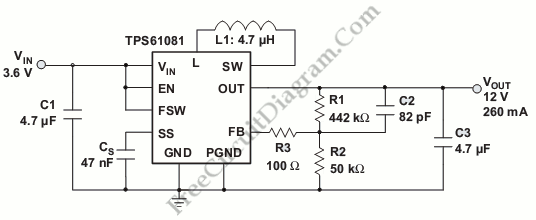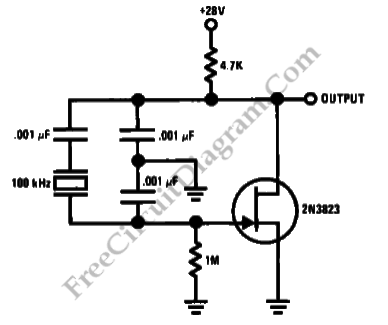## Lamp Dimmer using 555IC PWM – Video Tutorial

This video is a tutorial of lamp dimmer using PWM. This video shows the use of PWM for lamp dimmer. In this video, the PWM kit uses a 555 IC. The brightness of the lamp is dimmed by changing the pulse. If we up the pulse slowly, the intensity of the lamp getting brighter. Here is the video :

## Versatile Boost Converter with TPS6108xThere are two types of highly integrated boost converters, the TPS61081 and  TPS61080.  Both of them require low input voltage of 2.5V and the output is adjustable up to 27 V. The TPS61080’s current limit rating of the integrated power switches is 0.5 A and 1.3 A for TPS61081. Here is the circuit : It is useful for industrial application […]

## Stable Low Frequency Crystal OscillatorThis is a Colpitts-crystal oscillator circuit. It is suitable for low frequency crystal oscillator circuits. Using the 2N3823 JFET, this circuit has excellent stability because the temperature will not vary the 2N3823 JFET circuit loading. Here is the circuit : [Source: National Semiconductor Application Note]

## DC Motor Speed Control – Video Tutorial

This is a video tutorial of DC motor control. This video shows how to control the DC motor speed. In this video, speed of the DC motor is controlled by changing the power supply. Increasing speed can be done by increasing the voltage, and decreasing the voltage will decrease the speed of DC motor. Here is the video : To […]

## Logic Probe – Video Tutorial

This is a video tutorial of logic probe or logic analyzer. This video shows what is logic probe and how can the logic probe analyzes. There are two main operation in the logic probe work, first is timing analyze and second is state analysis. In this video also shows the different between them. Beside that, this video shows the sequential […]